# Exam 1 Review Exam 1 n Exam 1

• Slides: 50Exam 1 ReviewExam 1 n Exam 1 today at 6: 55 pm-8: 45 pm. n Honor Code: n n Open book, open notes, open slides, no use of compilers, no communication with others. You must only submit *your own* answers. Type into Submitty (like Quizzes). 2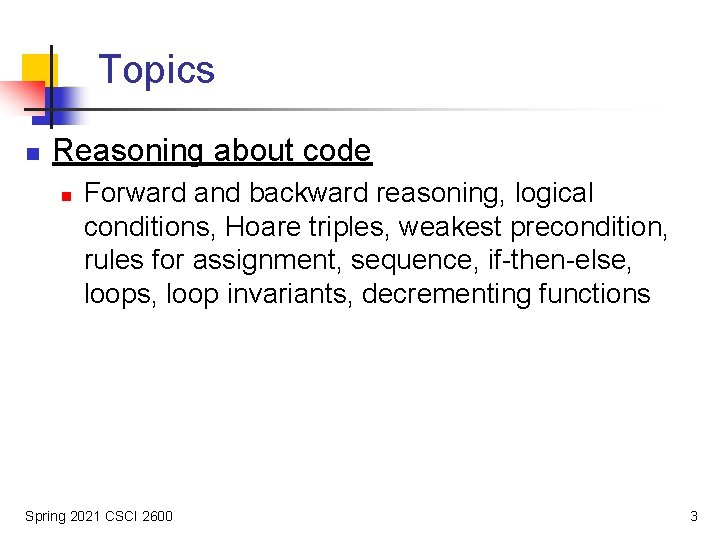Topics n Reasoning about code n Forward and backward reasoning, logical conditions, Hoare triples, weakest precondition, rules for assignment, sequence, if-then-else, loops, loop invariants, decrementing functions Spring 2021 CSCI 2600 3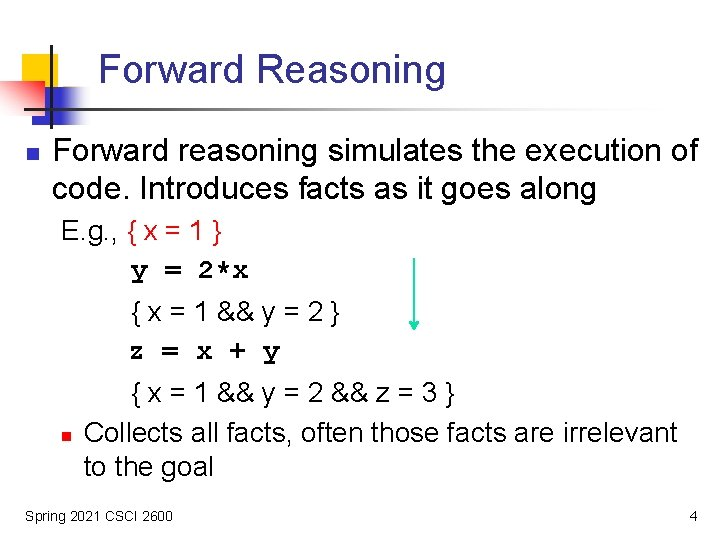Forward Reasoning n Forward reasoning simulates the execution of code. Introduces facts as it goes along E. g. , { x = 1 } y = 2*x { x = 1 && y = 2 } z = x + y n { x = 1 && y = 2 && z = 3 } Collects all facts, often those facts are irrelevant to the goal Spring 2021 CSCI 2600 4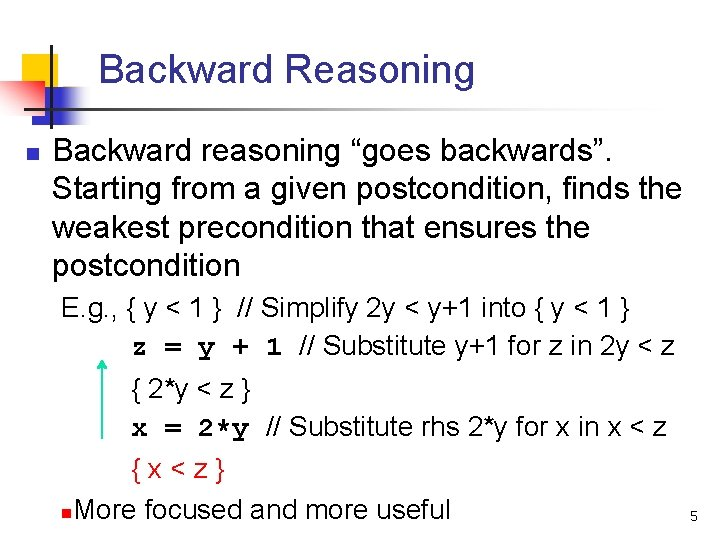Backward Reasoning n Backward reasoning “goes backwards”. Starting from a given postcondition, finds the weakest precondition that ensures the postcondition E. g. , { y < 1 } // Simplify 2 y < y+1 into { y < 1 } z = y + 1 // Substitute y+1 for z in 2 y < z { 2*y < z } x = 2*y // Substitute rhs 2*y for x in x < z {x<z} n. More focused and more useful 5Condition Strength n n “P is stronger than Q” means “P implies Q” “P is stronger than Q” means “P guarantees no less than Q” n n Values satisfying P also satisfy Q n n n E. g. , x>0 is stronger than x>-1 E. g. , values satisfying x>0 also satisfy x>-1 Stronger means more specific Weaker means more general 6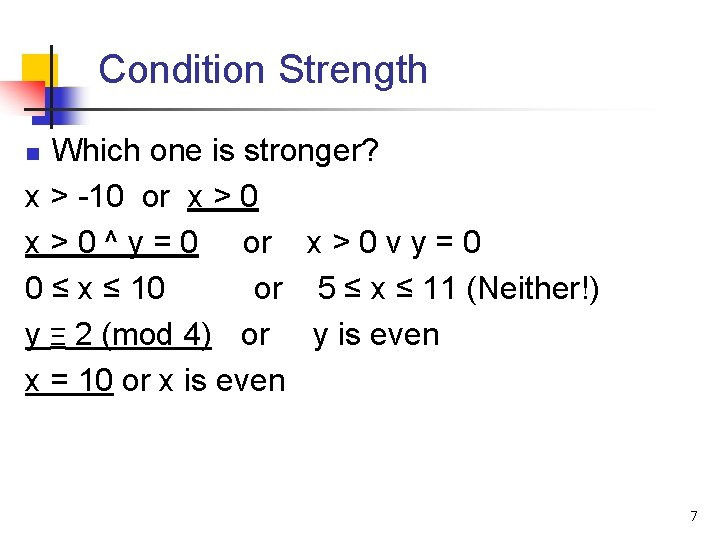Condition Strength Which one is stronger? x > -10 or x > 0 ^ y = 0 or x > 0 v y = 0 0 ≤ x ≤ 10 or 5 ≤ x ≤ 11 (Neither!) y Ξ 2 (mod 4) or y is even x = 10 or x is even n 7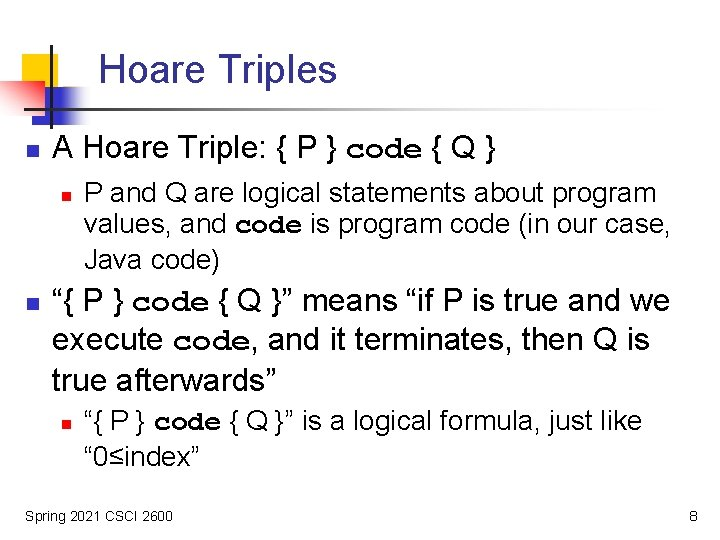Hoare Triples n A Hoare Triple: { P } code { Q } n n P and Q are logical statements about program values, and code is program code (in our case, Java code) “{ P } code { Q }” means “if P is true and we execute code, and it terminates, then Q is true afterwards” n “{ P } code { Q }” is a logical formula, just like “ 0≤index” Spring 2021 CSCI 2600 8Examples of Hoare Triples { x>0 } x++ { x>1 } is true { x>0 } x++ { x>-1 } is true { x≥ 0 } x++ { x>1 } is false. Why? {x>0} x++ {x>0} is ? ? {x<0} x++ {x<0} is ? ? {x=a} if (x < 0) x=-x { x = | a | } is ? ? {x=y} x=x+3 {x=y} is ? ? Spring 2021 CSCI 2600 9Rules for Backward Reasoning: Assignment // precondition: ? ? x = expression // postcondition: Q Rule: the weakest precondition = Q, with all occurrences of x in Q replaced by expression More formally: wp(“x=expression; ”, Q) = Q with all occurrences of x replaced by expression 10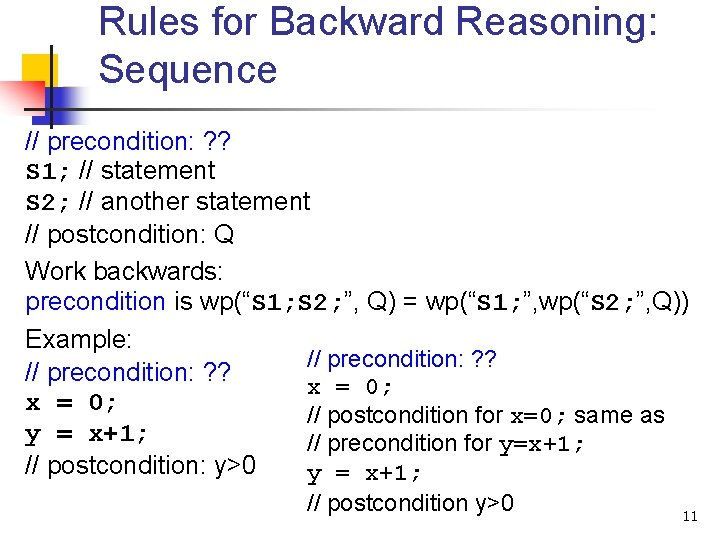Rules for Backward Reasoning: Sequence // precondition: ? ? S 1; // statement S 2; // another statement // postcondition: Q Work backwards: precondition is wp(“S 1; S 2; ”, Q) = wp(“S 1; ”, wp(“S 2; ”, Q)) Example: // precondition: ? ? x = 0; // postcondition for x=0; same as y = x+1; // precondition for y=x+1; // postcondition: y>0 y = x+1; // postcondition y>0 11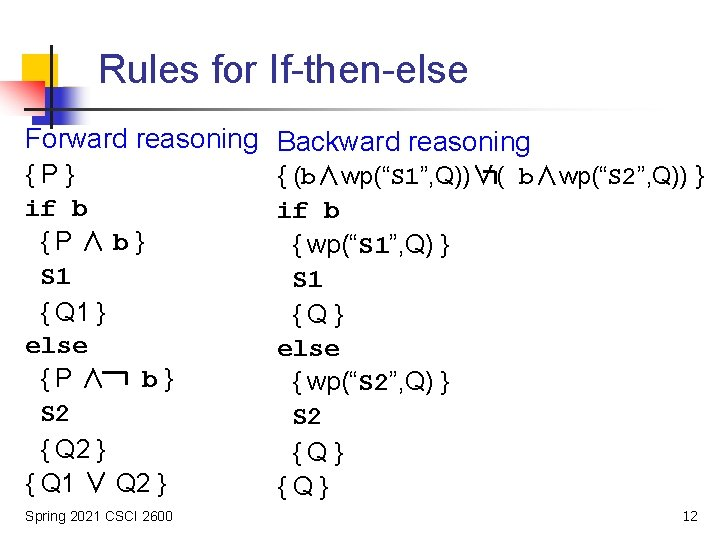Rules for If-then-else Forward reasoning Backward reasoning {P} if b {P∧b} S 1 { Q 1 } else {P∧ b} S 2 { Q 2 } { Q 1 ∨ Q 2 } Spring 2021 CSCI 2600 { (b∧wp(“S 1”, Q))∨( b∧wp(“S 2”, Q)) } if b { wp(“S 1”, Q) } S 1 {Q} else { wp(“S 2”, Q) } S 2 {Q} 12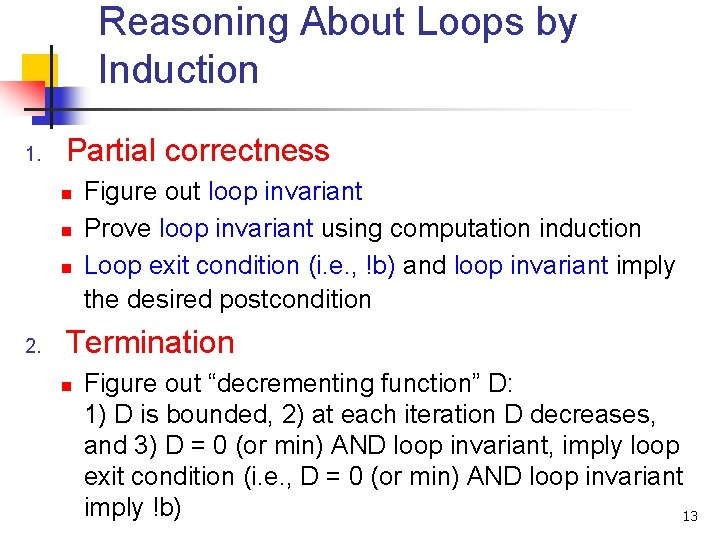Reasoning About Loops by Induction 1. Partial correctness n n n 2. Figure out loop invariant Prove loop invariant using computation induction Loop exit condition (i. e. , !b) and loop invariant imply the desired postcondition Termination n Figure out “decrementing function” D: 1) D is bounded, 2) at each iteration D decreases, and 3) D = 0 (or min) AND loop invariant, imply loop exit condition (i. e. , D = 0 (or min) AND loop invariant imply !b) 13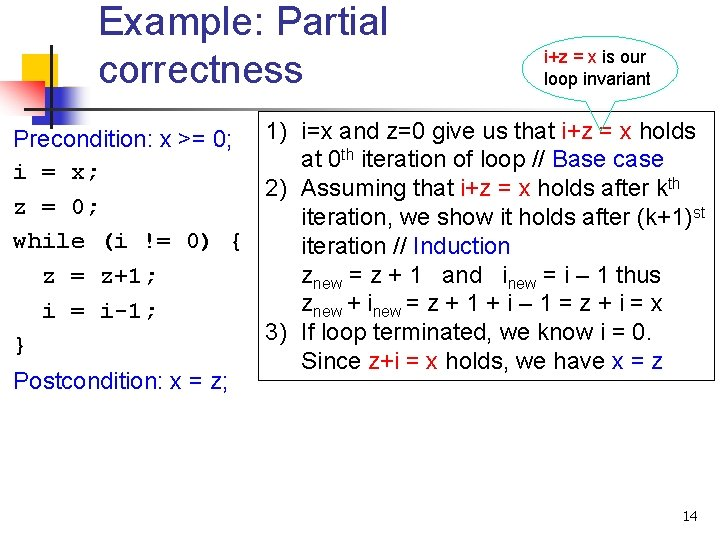Example: Partial correctness i+z = x is our loop invariant Precondition: x >= 0; 1) i=x and z=0 give us that i+z = x holds th iteration of loop // Base case at 0 i = x; 2) Assuming that i+z = x holds after kth z = 0; iteration, we show it holds after (k+1)st while (i != 0) { iteration // Induction znew = z + 1 and inew = i – 1 thus z = z+1; znew + inew = z + 1 + i – 1 = z + i = x i = i-1; 3) If loop terminated, we know i = 0. } Since z+i = x holds, we have x = z Postcondition: x = z; 14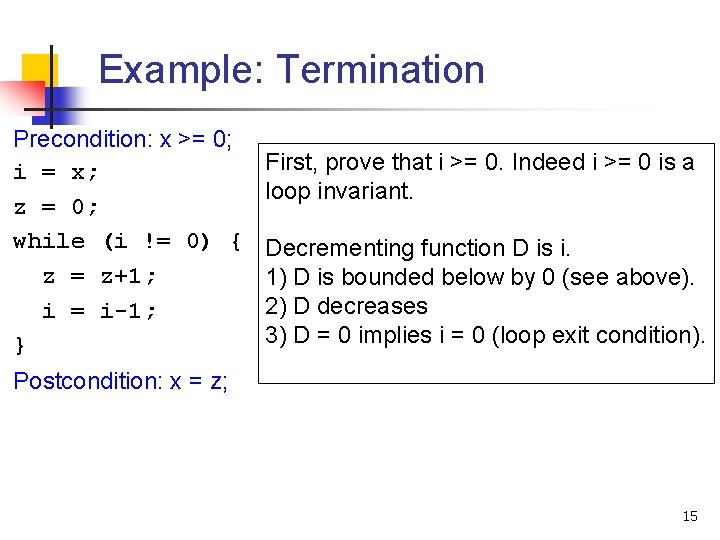Example: Termination Precondition: x >= 0; i = x; z = 0; while (i != 0) { z = z+1; i = i-1; } First, prove that i >= 0. Indeed i >= 0 is a loop invariant. Decrementing function D is i. 1) D is bounded below by 0 (see above). 2) D decreases 3) D = 0 implies i = 0 (loop exit condition). Postcondition: x = z; 15Reasoning About Loops { P } while (b) S { Q } 16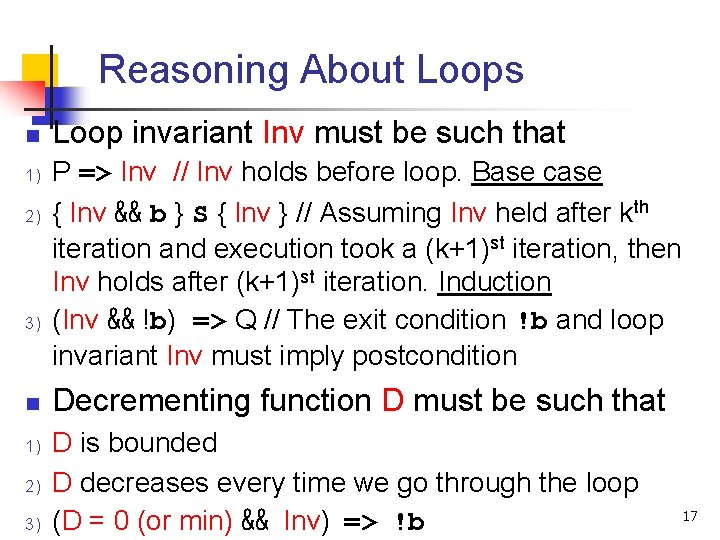Reasoning About Loops n 1) 2) 3) Loop invariant Inv must be such that P => Inv // Inv holds before loop. Base case { Inv && b } S { Inv } // Assuming Inv held after kth iteration and execution took a (k+1)st iteration, then Inv holds after (k+1)st iteration. Induction (Inv && !b) => Q // The exit condition !b and loop invariant Inv must imply postcondition Decrementing function D must be such that D is bounded D decreases every time we go through the loop (D = 0 (or min) && Inv) => !b 17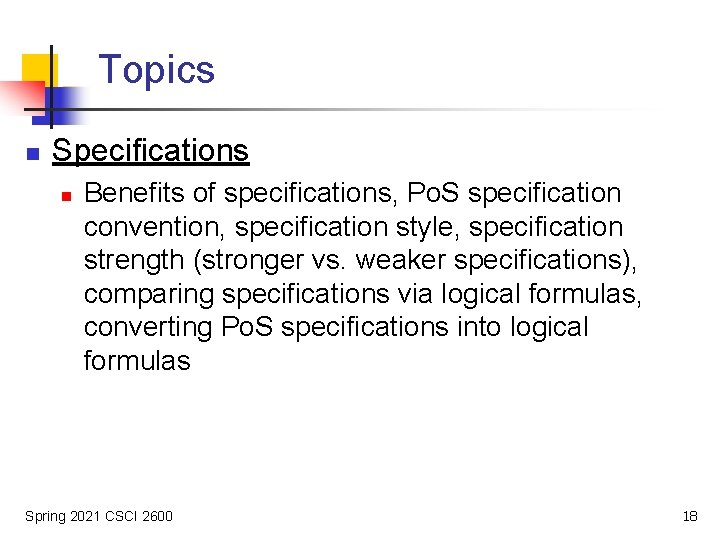Topics n Specifications n Benefits of specifications, Po. S specification convention, specification style, specification strength (stronger vs. weaker specifications), comparing specifications via logical formulas, converting Po. S specifications into logical formulas Spring 2021 CSCI 2600 18Specifications n A specification consists of a precondition and a postcondition n n Precondition: conditions that hold before method executes Postcondition: conditions that hold after method finished execution (if precondition held!) 19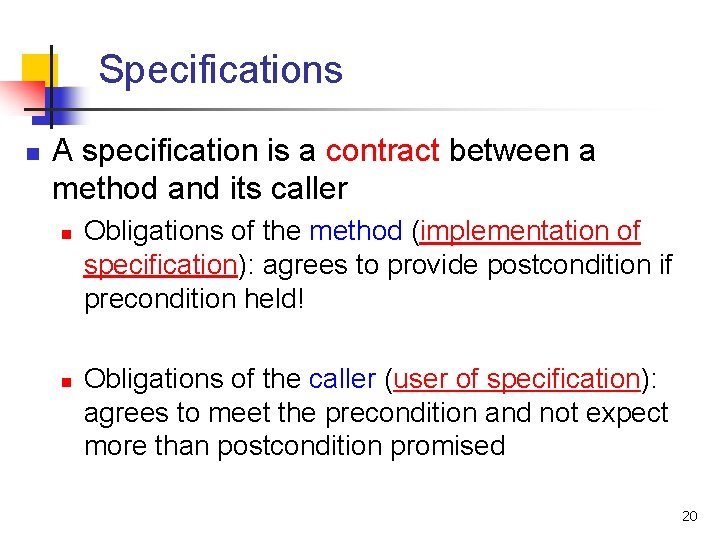Specifications n A specification is a contract between a method and its caller n n Obligations of the method (implementation of specification): agrees to provide postcondition if precondition held! Obligations of the caller (user of specification): agrees to meet the precondition and not expect more than postcondition promised 20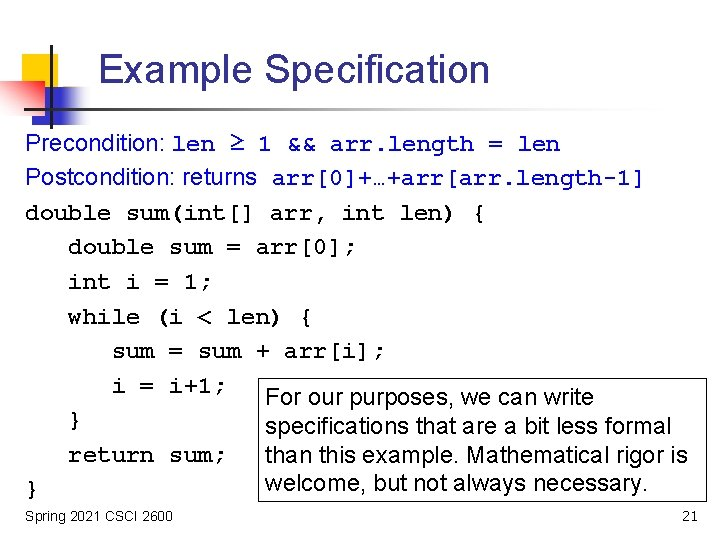Example Specification Precondition: len ≥ 1 && arr. length = len Postcondition: returns arr+…+arr[arr. length-1] double sum(int[] arr, int len) { double sum = arr; int i = 1; while (i < len) { sum = sum + arr[i]; i = i+1; For our purposes, we can write } specifications that are a bit less formal return sum; than this example. Mathematical rigor is welcome, but not always necessary. } Spring 2021 CSCI 2600 21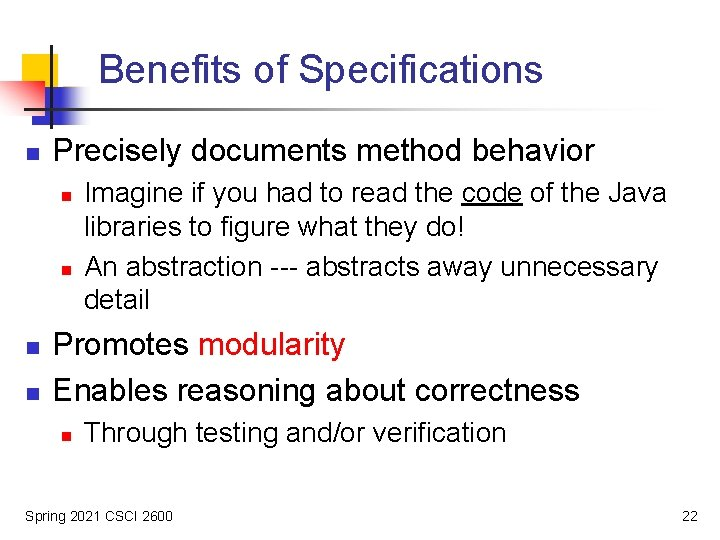Benefits of Specifications n Precisely documents method behavior n n Imagine if you had to read the code of the Java libraries to figure what they do! An abstraction --- abstracts away unnecessary detail Promotes modularity Enables reasoning about correctness n Through testing and/or verification Spring 2021 CSCI 2600 22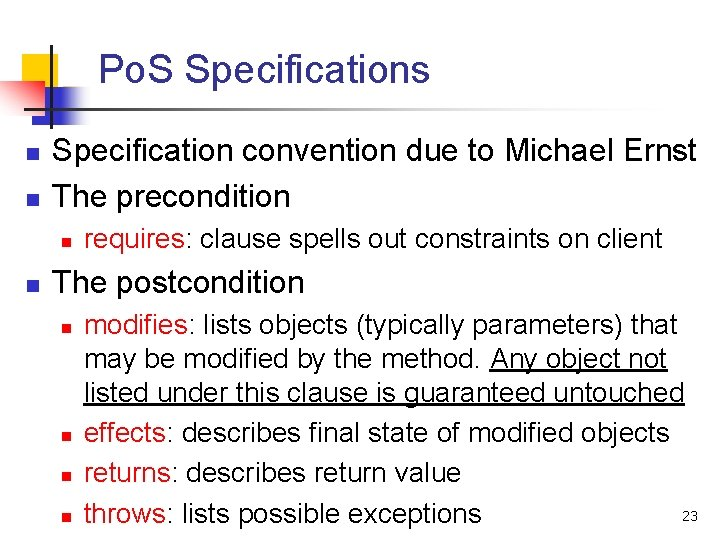Po. S Specifications n n Specification convention due to Michael Ernst The precondition n n requires: clause spells out constraints on client The postcondition n n modifies: lists objects (typically parameters) that may be modified by the method. Any object not listed under this clause is guaranteed untouched effects: describes final state of modified objects returns: describes return value 23 throws: lists possible exceptionsExample static List<Integer> list. Add(List<Integer> lst 1, List<Integer> lst 2) requires: lst 1, lst 2 are non-null. lst 1 and lst 2 are same size. modifies: none effects: none returns: a new list of the same size, whose i-th element is the sum of the i-th elements of lst 1 and lst 2 static List<Integer> list. Add(List<Integer> lst 1, List<Integer> lst 2) { List<Integer> res = new Array. List<Integer>(); for (int i = 0; i < lst 1. size(); i++) res. add(lst 1. get(i) + lst 2. get(i)); return res; } Spring 2021 CSCI 2600 24Another Example static void list. Add 2(List<Integer> lst 1, List<Integer> lst 2) requires: lst 1, lst 2 are non-null. lst 1 and lst 2 are same size. modifies: lst 1 effects: i-th element of lst 1 is replaced with the sum of i-th elements of lst 1 and lst 2 returns: none static void list. Add(List<Integer> lst 1, List<Integer> lst 2) { for (int i = 0; i < lst 1. size(); i++) { lst 1. set(i, lst 1. get(i) + lst 2. get(i)); } } Spring 2021 CSCI 2600 25Specification Style n A method is called for its side effects (effects clause) or its return value (returns clause) n n It is bad style to have both effects and return There are exceptions n n Main point of spec is to be helpful n n n E. g. , Hash. Map. put returns the previous value Being overly formal may not help Being too informal does not help either If spec turns too complex: redesign. Better to 26 simplify code than document complexity!What’s Wrong? static void uniquefy(List<Integer> lst) requires: ? modifies: ? effects: ? returns: ? static void uniquefy(List<Integer> lst) { for (int i = 0; i < lst. size()-1; i++) if (lst. get(i) == lst. get(i+1)) lst. remove(i); } Spring 2021 CSCI 2600 27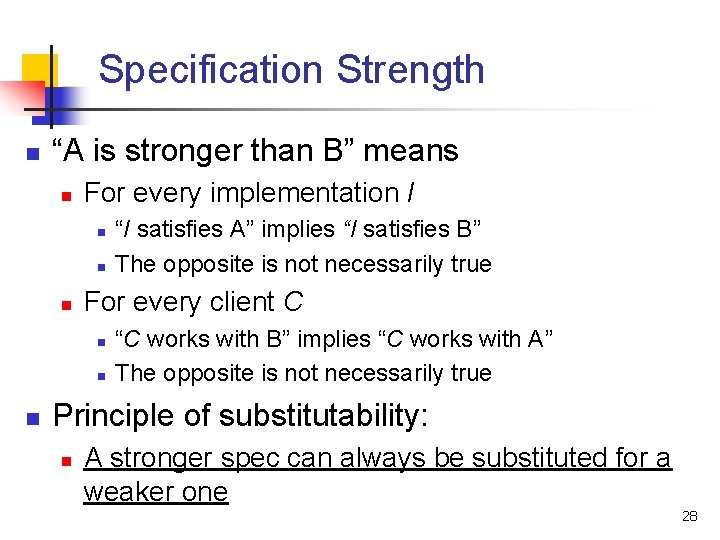Specification Strength n “A is stronger than B” means n For every implementation I n n n For every client C n n n “I satisfies A” implies “I satisfies B” The opposite is not necessarily true “C works with B” implies “C works with A” The opposite is not necessarily true Principle of substitutability: n A stronger spec can always be substituted for a weaker one 28Why Care About Specification Strength? n Because of substitutability! n Principle of substitutability n n A stronger specification can always be substituted for a weaker one I. e. , an implementation that conforms to a stronger specification can be used in a client that expects a weaker specification Spring 2021 CSCI 2600 29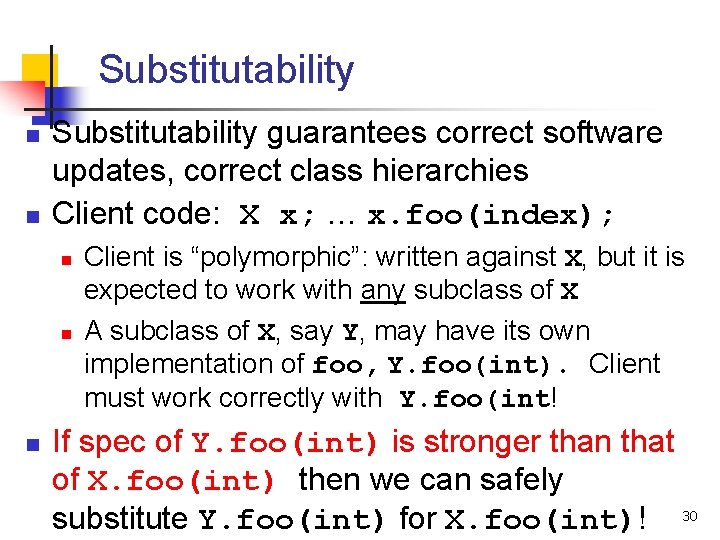Substitutability n n Substitutability guarantees correct software updates, correct class hierarchies Client code: X x; … x. foo(index); n n n Client is “polymorphic”: written against X, but it is expected to work with any subclass of X A subclass of X, say Y, may have its own implementation of foo, Y. foo(int). Client must work correctly with Y. foo(int! If spec of Y. foo(int) is stronger than that of X. foo(int) then we can safely substitute Y. foo(int) for X. foo(int)! 30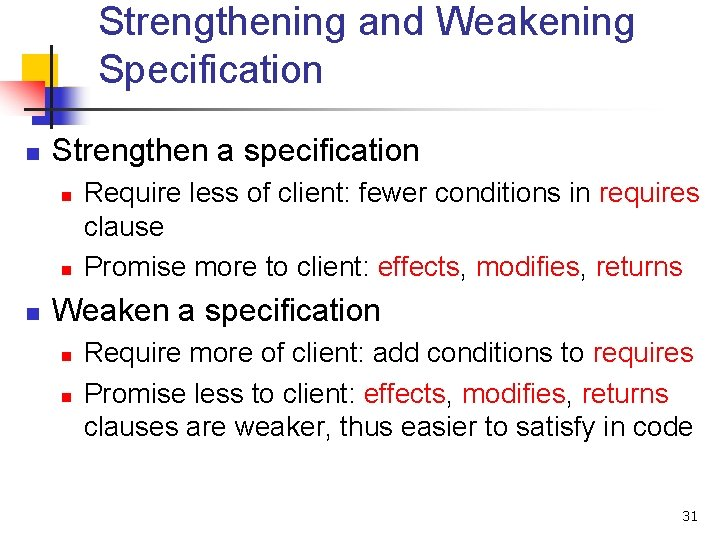Strengthening and Weakening Specification n Strengthen a specification n Require less of client: fewer conditions in requires clause Promise more to client: effects, modifies, returns Weaken a specification n n Require more of client: add conditions to requires Promise less to client: effects, modifies, returns clauses are weaker, thus easier to satisfy in code 31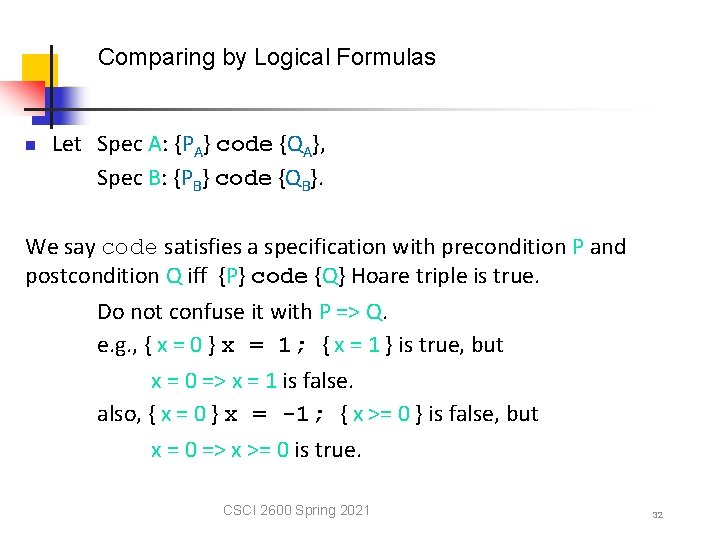Comparing by Logical Formulas n Let Spec A: {PA} code {QA}, Spec B: {PB} code {QB}. We say code satisfies a specification with precondition P and postcondition Q iff {P} code {Q} Hoare triple is true. Do not confuse it with P => Q. e. g. , { x = 0 } x = 1; { x = 1 } is true, but x = 0 => x = 1 is false. also, { x = 0 } x = -1; { x >= 0 } is false, but x = 0 => x >= 0 is true. CSCI 2600 Spring 2021 32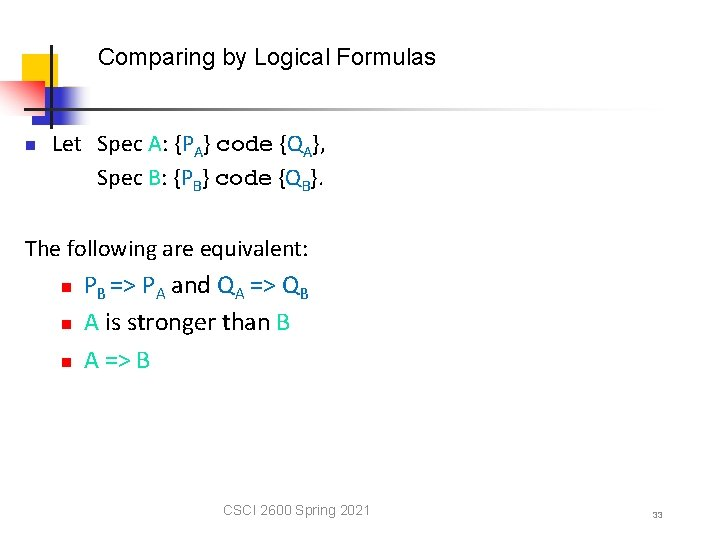Comparing by Logical Formulas n Let Spec A: {PA} code {QA}, Spec B: {PB} code {QB}. The following are equivalent: n n n PB => PA and QA => QB A is stronger than B A => B CSCI 2600 Spring 2021 33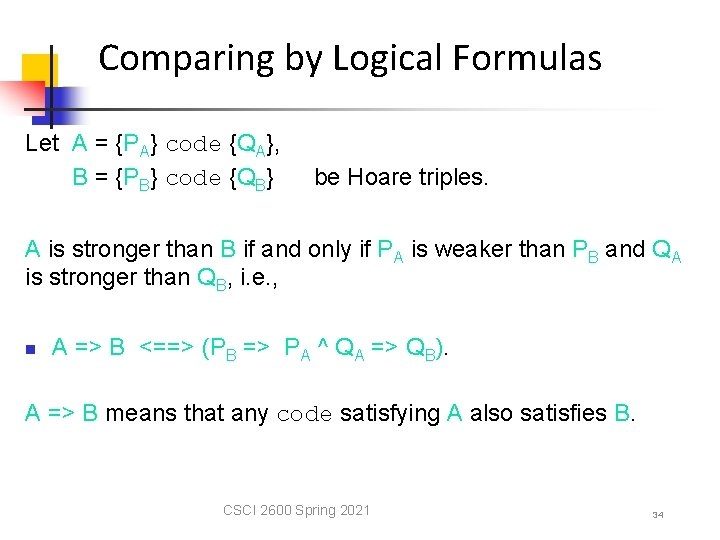Comparing by Logical Formulas Let A = {PA} code {QA}, B = {PB} code {QB} be Hoare triples. A is stronger than B if and only if PA is weaker than PB and QA is stronger than QB, i. e. , n A => B <==> (PB => PA ^ QA => QB). A => B means that any code satisfying A also satisfies B. CSCI 2600 Spring 2021 34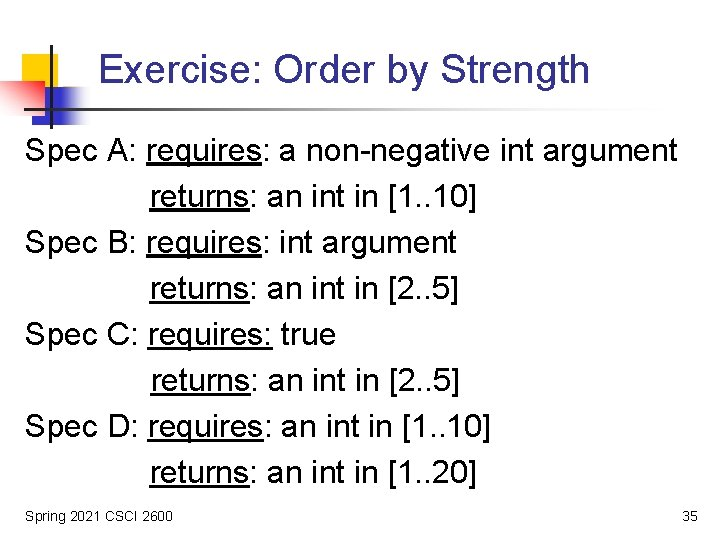Exercise: Order by Strength Spec A: requires: a non-negative int argument returns: an int in [1. . 10] Spec B: requires: int argument returns: an int in [2. . 5] Spec C: requires: true returns: an int in [2. . 5] Spec D: requires: an int in [1. . 10] returns: an int in [1. . 20] Spring 2021 CSCI 2600 35Converting Po. S Specs into Logical Formulas Po. S specification requires: R modifies: M effects: E // absorbs throws, returns and effects Spec is equivalent to the following logical formula: {R} code { E ^ (nothing but M is modified)} n Step 1: absorb throws and returns into effects E Step 2: {R} code {( E ^(nothing but M is modified)} Spring 2021 CSCI 2600 36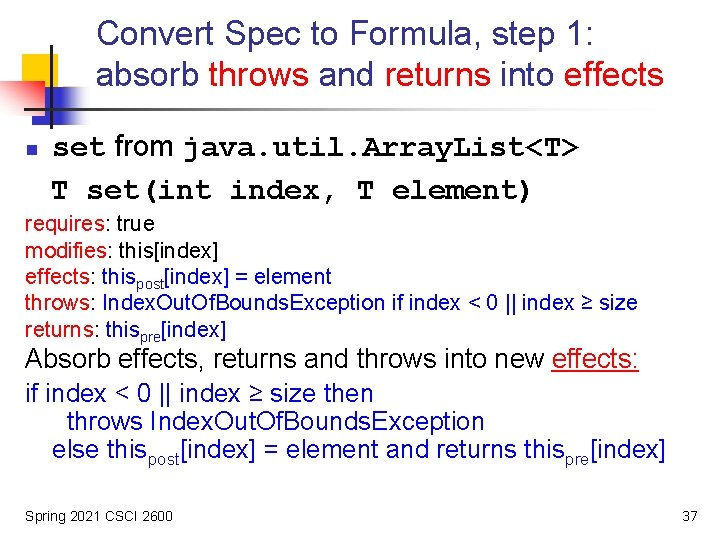Convert Spec to Formula, step 1: absorb throws and returns into effects n set from java. util. Array. List<T> T set(int index, T element) requires: true modifies: this[index] effects: thispost[index] = element throws: Index. Out. Of. Bounds. Exception if index < 0 || index ≥ size returns: thispre[index] Absorb effects, returns and throws into new effects: if index < 0 || index ≥ size then throws Index. Out. Of. Bounds. Exception else thispost[index] = element and returns thispre[index] Spring 2021 CSCI 2600 37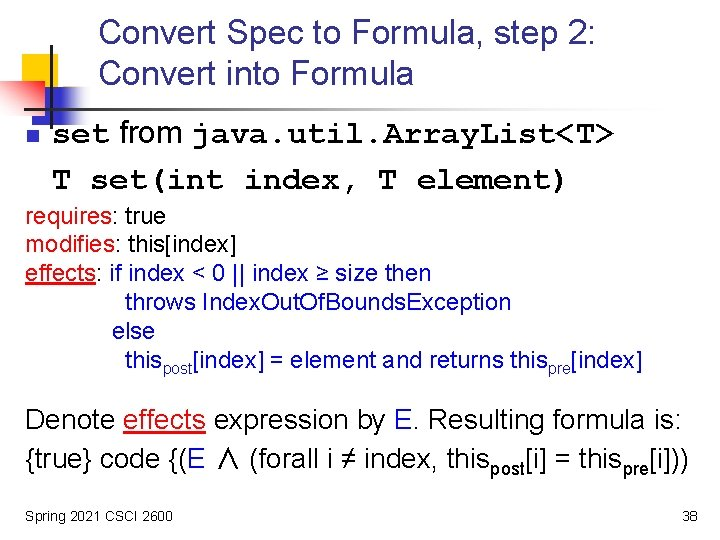Convert Spec to Formula, step 2: Convert into Formula n set from java. util. Array. List<T> T set(int index, T element) requires: true modifies: this[index] effects: if index < 0 || index ≥ size then throws Index. Out. Of. Bounds. Exception else thispost[index] = element and returns thispre[index] Denote effects expression by E. Resulting formula is: {true} code {(E ∧ (forall i ≠ index, thispost[i] = thispre[i])) Spring 2021 CSCI 2600 38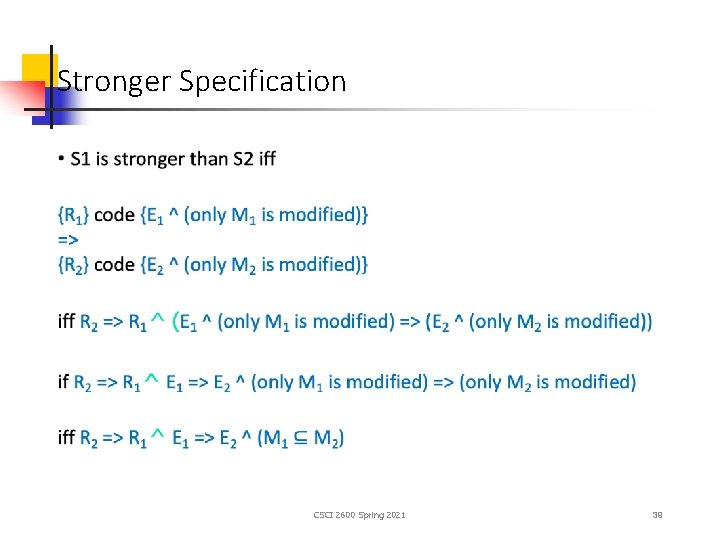Stronger Specification CSCI 2600 Spring 2021 39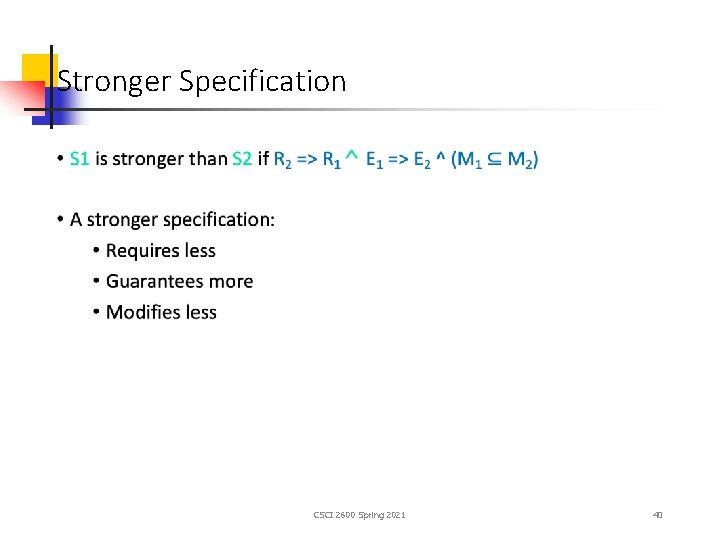Stronger Specification CSCI 2600 Spring 2021 40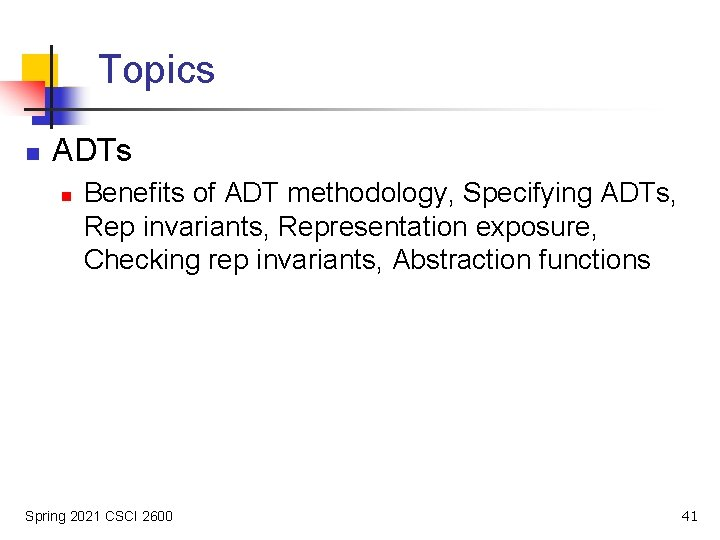Topics n ADTs n Benefits of ADT methodology, Specifying ADTs, Rep invariants, Representation exposure, Checking rep invariants, Abstraction functions Spring 2021 CSCI 2600 41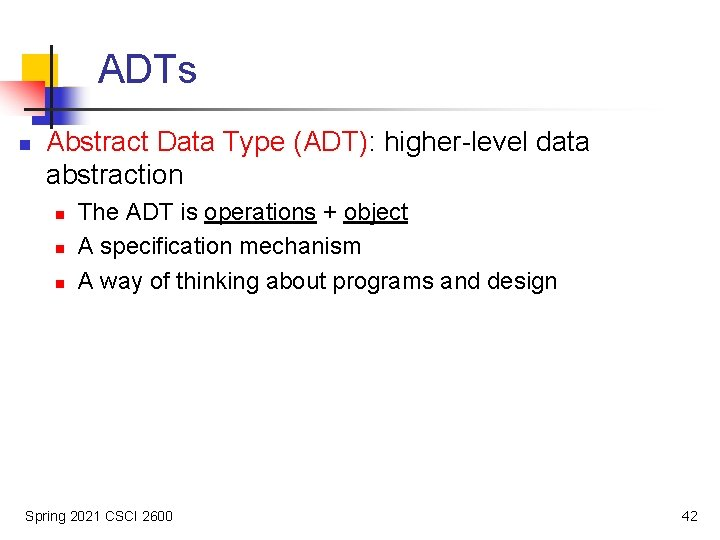ADTs n Abstract Data Type (ADT): higher-level data abstraction n The ADT is operations + object A specification mechanism A way of thinking about programs and design Spring 2021 CSCI 2600 42An ADT Is a Set of Operations n n Operations operate on data representation ADT abstracts from organization to meaning of data ADT abstracts from structure to use Data representation does not matter! class Roint { � float x, y; } n n class Point { � float r, theta; } Instead, think of a type as a set of operations: create, x(), y(), r(), theta(). Force clients to call operations to access data Spring 2021 CSCI 2600 43Specifying an ADT immutable class Type. Name 1. overview 2. abstract fields 3. creators 4. observers 5. producers 6. mutators 1. overview 2. abstract fields 3. creators 4. observers 5. producers (rare!) 6. mutators Spring 2021 CSCI 2600 44Connecting Implementation to Specification n Representation invariant: Object boolean n Indicates whether data representation is wellformed. Only well-formed representations are meaningful Defines the set of valid values Abstraction function: Object abstract value n What the data structure really means n n E. g. , array [2, 3, -1] represents –x 2 + 3 x + 2 How the data structure is to be interpreted Spring 2021 CSCI 2600 45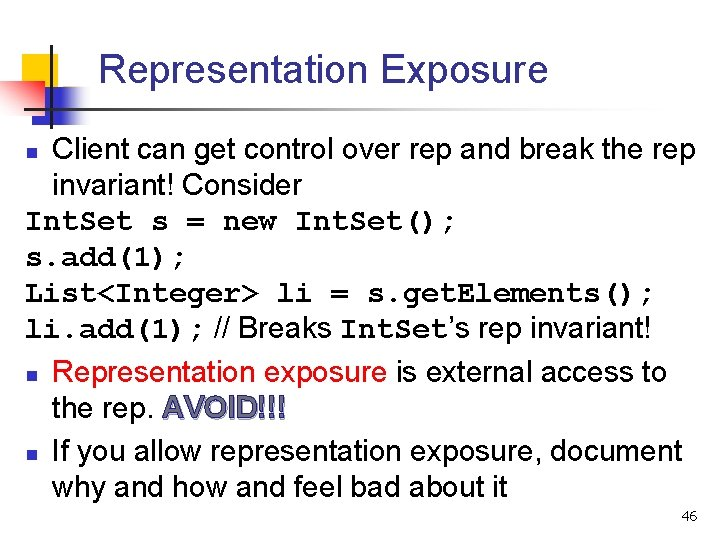Representation Exposure Client can get control over rep and break the rep invariant! Consider Int. Set s = new Int. Set(); s. add(1); List<Integer> li = s. get. Elements(); li. add(1); // Breaks Int. Set’s rep invariant! n Representation exposure is external access to the rep. AVOID!!! n If you allow representation exposure, document why and how and feel bad about it n 46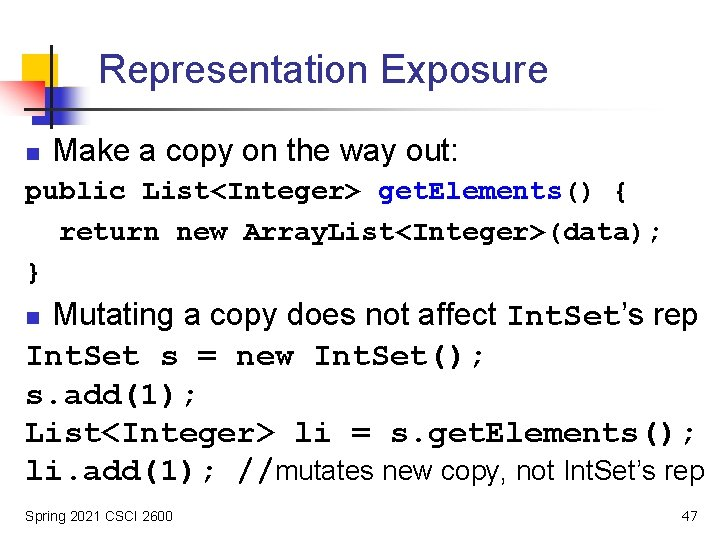Representation Exposure n Make a copy on the way out: public List<Integer> get. Elements() { return new Array. List<Integer>(data); } Mutating a copy does not affect Int. Set’s rep Int. Set s = new Int. Set(); s. add(1); List<Integer> li = s. get. Elements(); li. add(1); //mutates new copy, not Int. Set’s rep n Spring 2021 CSCI 2600 47Representation Exposure n Make a copy on the way in too: public Int. Set(Array. List<Integer> elts) { data = new Array. List<Integer>(elts); … } n Why? Spring 2021 CSCI 2600 48Abstraction Function n Abstraction function allows us to reason about correctness of the implementation Abstract value Abstract operation: Abstract value’ � AF: Concrete object � Spring 2021 CSCI 2600 � Concrete operation (i. e. , our implementation of operation): AF: Concrete object’ � 49Int. Set Example { 1, 2, 3 } abstract remove(1): this – { 1 } { 2, 3 } � � AF: Concrete remove(1) [2, 1, 1, 2, 3] � Creating concrete object: Establish rep invariant Establish abstraction function Spring 2021 CSCI 2600 [2, 2, 3] � After every operations: Maintains rep invariant Maintains abstraction function 50